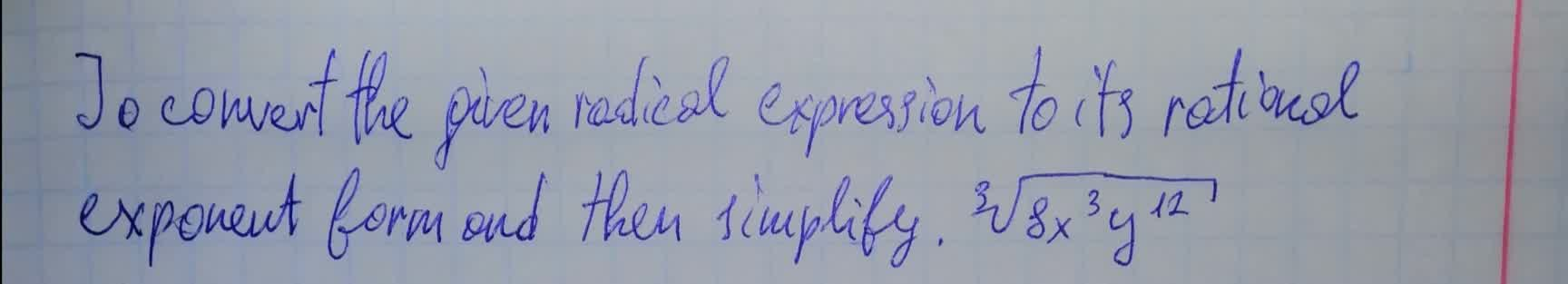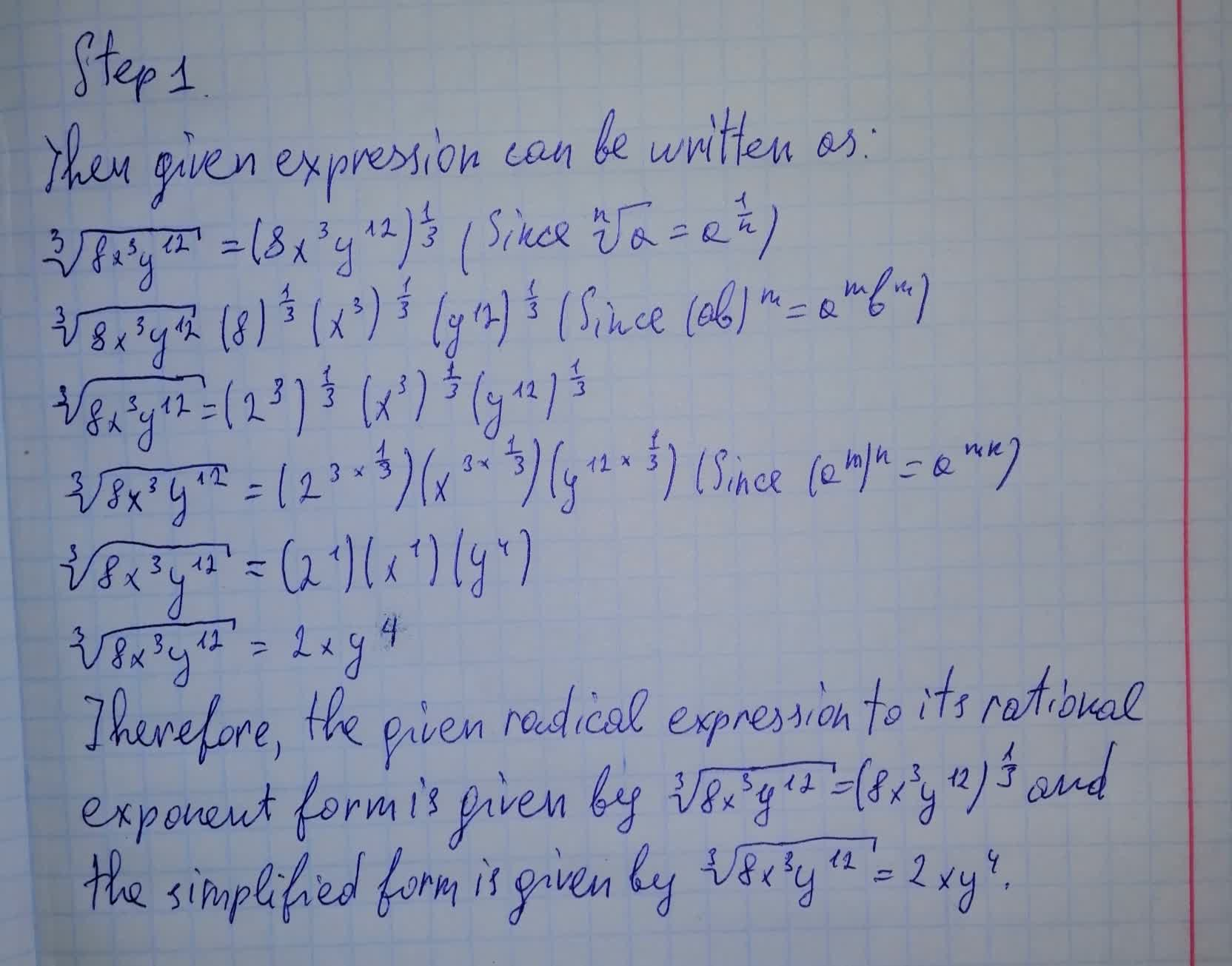To convert the given radical expression to its rational exponent form and then simplifyDofotheroU 2021-08-10 Answered
To convert the given radical expression to its rational exponent form and then simplify• Questions are typically answered in as fast as 30 minutes

Solve your problem for the price of one coffee

• Math expert for every subject
• Pay only if we can solve itensojadasHNot exactly what you’re looking for?Vasquez

Step 1

Then given expression can be written as:

$$\sqrt{8x^3y^{12}}=(8x^3 y^{12})^{\frac 13} \ \ \ (\text{Since } \sqrt[n]{a}=a^{\frac 1n})$$

$$\sqrt{8x^3y^{12}} (8)^{\frac 13} (x^3)^{\frac 13} (y^{12})^{\frac 13} \ \ \ \ (Since (ab)^m=a^m b^m)$$

$$\sqrt{8x^3 y^{12}}=(2^3)^{\frac 13} (x^3)^{\frac 13} (y^{12})^{\frac 13}$$

$$\sqrt{8x^3y^{12}}=(2^{3 \times \frac 13})(x^{3 \times \frac 13}) (y^{12 \times \frac 13}) \ \ \ (\text{Since } (a^m)^n=a^{mn})$$

$$\sqrt{8x^3y^{12}}=(2^1)(x^1)(y^4)$$

$$\sqrt{8x^3y^{12}}=2 x y^4$$

Therefore, the given radical expression to its rational exponent form is given by $$\sqrt{8x^3y^{12}}=(8x^3 y^{12})^{\frac 13}$$ and the simplified form is given by $$\sqrt{8x^3y^{12}}=2xy^4$$